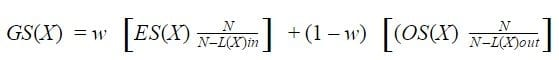Select Page

Since my first job, I have always used spreadsheets as a tool to complete my tasks. As I have always worked in small companies, Microsoft Excel was not an option because it is not free. On the other hand, Google Sheets allows you to share any spreadsheet and edit simultaneously. Moreover, it is for free, so Google Sheets ended up being the best option.

In my first job, my team and I had some important information held in a few key spreadsheets like KPIs or CRM data. But we also had a lot less important data held in other spreadsheets. I’m now working at Sheetgo and we are in the process of accelerated growth. Sheetgo has an Add-on for Google Sheets that allows you to connect data between different spreadsheets and update them automatically.

We are pioneers in connecting data in spreadsheets and we want to better understand these connections. So we’ve begun to study the relevance of each spreadsheet in order to make sure we’re controlling our data well and to know which sheets are irrelevant and can be removed. Moreover, this measurement is a scoring spreadsheet feature that we hope to provide to our customers coming soon.

### Connected spreadsheets

Sheetgo gives users the ability to connect their spreadsheets. Our hypothesis is that the importance of each sheet depends on their connections with others spreadsheets. Why? If one spreadsheet receives or sends data to five, ten or twenty it is clear that it will be more important than other that neither receive nor send data.

We’ve plotted out the systems created by several of our users and we see patterns like the following:Where each dot is a spreadsheet and the width of arrows measure the number of tabs that are shared.

### Scoring spreadsheet: How to measure each one

Each spreadsheet can import data from others or export data to others. If one spreadsheet imports a lot of data, it is important to the organization because it is really useful for monitoring data. On the other hand, a spreadsheet that exports data to many others is also important because this spreadsheet is likely a central database.

We decided to do a spreadsheet ranking based on the capacity of received data of each spreadsheet. To measure this we developed the following formula based on Page Rank from Google:Where “N” is total connections in the spreadsheet system, and “d” is a factor of connectivity. If d is higher, the score of a none connected spreadsheet is lower. Therefore, the “entry score” of any worksheet (ES(X)) depends on the input score of each worksheet from which it receives data. L(Y)out is the number of connections out of Y to others spreadsheets.

At the same time we can measure the capacity of send data of each worksheet:Finally, in order to get a global score of X (GS(X)) for each spreadsheet, we will sum ES(X) and OS(X) and we will multiply them by “w”. W is used to give more weight to the capacity to either send or receive data.### Case study and conclusions

In the next image you can see an example from one of our users:It seems that mathematical formulas work well, at least in this example and all that we have already analyzed. Anyway, what information can we extract? First, we can define which spreadsheets are the core of our data system. They will be those with more “Output Power“. Second, we will consider spreadsheets that are useful for monitoring data like KPIs for instance. Finally, we will know the global punctuation of each spreadsheet.

Ranking your spreadsheets will help you shape your data management procedures and help you ensure that the most important spreadsheets are up to date. It will also help you to know what scoring spreadsheet might be unnecessary and finally allow us to analyze the possibility of improving the efficiency of our spreadsheet system(s).

Share This